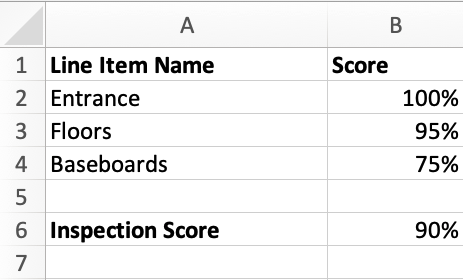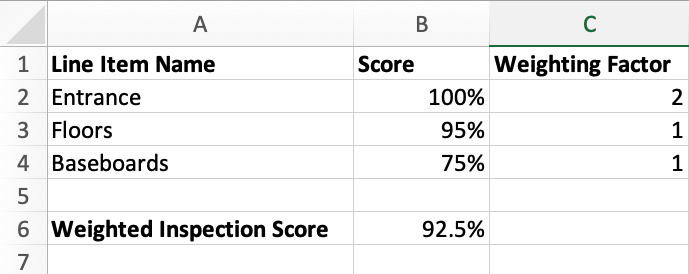# How Are Inspection Scores Calculated?

Inspection scores are calculated based on the weight you've assigned each line item. Here are a couple examples of how we calculate the final score, depending on whether the line item has equal or different weighting factors.

## Inspections with equal weighting factors

If an inspection contains line items that are all equally weighted, then the score is simply the average of the scored line items. Here's an example:## Inspections with different weighting factors

The score for an inspection is based on a weighted average of the line items. By changing the "weight," you can make some items count more towards the overall score.

It is important to remember that the Weighting Factor does not need to add up to 100.

For example, say you have an inspection with three items, all using a percentage rating:

• Entrance (This is the most important item)
• Floors
• Baseboards

Because the Entrance is the most important item, we assign it a weighting factor of 2. This makes it twice as important as other items.

```(100*2) + (95*1) + (75*1)
-----------------------
100*2 + 100*1 + 100*1
```

This would give you an overall score of 92.5%.